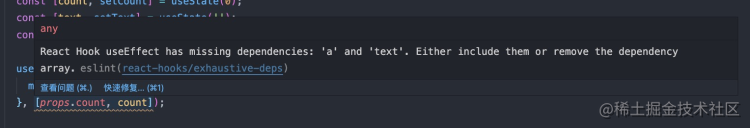# React Hooks 使用误区，驳官方文档

## 1. 不是所有的依赖都必须放到依赖数组中

``````function Demo(props) {
const [count, setCount] = useState(0);
const [text, setText] = useState('');
const [a, setA] = useState('');

useEffect(() => {
monitor(props.count, count, text, a);
}, [props.count, count]);

return (
<div>
<button
onClick={() => setCount(c => c + 1)}
>
click
</button>
<input value={text} onChange={e => setText(e.target.value)} />
<input value={a} onChange={e => setA(e.target.value)} />
</div>
)
}``````  useEffect(() => {
monitor(props.count, count, text, a);
}, [props.count, count, text, a]);

1. 不要使用 `eslint-plugin-react-hooks` 插件，或者可以选择性忽略该插件的警告。
2. 只有一种情况，需要把变量放到 deps 数组中，那就是当该变量变化时，需要触发 useEffect 函数执行。而不是因为 useEffect 中用到了这个变量！

## 2. deps 参数不能缓解闭包问题

``````function Demo() {
const [count, setCount] = useState(0);

useEffect(() => {
const timer = setTimeout(() => {
console.log(count)
}, 3000);
return () => {
clearTimeout(timer);
}
}, [])

return (
<button
onClick={() => setCount(c => c + 1)}
>
click
</button>
)
}

``````  useEffect(() => {
const timer = setTimeout(() => {
console.log(count)
}, 3000);
return () => {
clearTimeout(timer);
}
}, [count])

``````const [count, setCount] = useState(0);

// 通过 ref 来记忆最新的 count
const countRef = useRef(count);
countRef.current = count;

useEffect(() => {
const timer = setTimeout(() => {
console.log(countRef.current)
}, 3000);
return () => {
clearTimeout(timer);
}
}, [])

``````const countRef = useRef(count);
countRef.current = count;

### 2.1 正常情况下是不会有闭包问题的

``````const [a, setA] = useState(0);
const [b, setB] = useState(0);

const c = a + b;

useEffect(()=>{
console.log(a, b, c)
}, [a]);

useEffect(()=>{
console.log(a, b, c)
}, [b]);

useEffect(()=>{
console.log(a, b, c)
}, [c]);

### 2.2 延迟调用会存在闭包问题

1. 使用 setTimeout、setInterval、Promise.then 等
2. useEffect 的卸载函数
``````const getUsername = () => {
return new Promise((resolve, reject) => {
setTimeout(() => {
resolve('John');
}, 3000);
})
}

function Demo() {
const [count, setCount] = useState(0);

// setTimeout 会造成闭包问题
useEffect(() => {
const timer = setTimeout(() => {
console.log(count);
}, 3000);
return () => {
clearTimeout(timer);
}
}, [])

// setInterval 会造成闭包问题
useEffect(() => {
const timer = setInterval(() => {
console.log(count);
}, 3000);
return () => {
clearInterval(timer);
}
}, [])

// Promise.then 会造成闭包问题
useEffect(() => {
console.log(count);
});
}, [])

// useEffect 卸载函数会造成闭包问题
useEffect(() => {
return () => {
console.log(count);
}
}, []);

return (
<button
onClick={() => setCount(c => c + 1)}
>
click
</button>
)
}

1. 组件初始化，此时 `count = 0`
2. 执行 useEffect，此时 useEffect 的函数执行，JS 引用链记录了对 `count=0` 的引用关系
3. 点击 button，count 变化，但对之前的引用已经无能为力了

``````const [count, setCount] = useState(0);

// 通过 ref 来记忆最新的 count
const countRef = useRef(count);
countRef.current = count;

useEffect(() => {
const timer = setTimeout(() => {
console.log(countRef.current)
}, 3000);
return () => {
clearTimeout(timer);
}
}, [])

......

1. 只有变化时，需要重新执行 useEffect 的变量，才要放到 deps 中。而不是 useEffect 用到的变量都放到 deps 中。
2. 在有延迟调用场景时，可以通过 ref 来解决闭包问题。

## 3. 尽量不要用 useCallback

### 3.1 useCallback 大部分场景没有提升性能

useCallback 可以记住函数，避免函数重复生成，这样函数在传递给子组件时，可以避免子组件重复渲染，提高性能。

``````const someFunc = useCallback(()=> {
doSomething();
}, []);

return <ExpensiveComponent func={someFunc} />

``````const ExpensiveComponent = ({ func }) => {
return (
<div onClick={func}>
hello
</div>
)
}

``````const ExpensiveComponent = React.memo(({ func }) => {
return (
<div onClick={func}>
hello
</div>
)
})

### 3.2 useCallback 让代码可读性变差

``````const someFuncA = useCallback((d, g, x, y)=> {
doSomething(a, b, c, d, g, x, y);
}, [a, b, c]);

const someFuncB = useCallback(()=> {
someFuncA(d, g, x, y);
}, [someFuncA, d, g, x, y]);

useEffect(()=>{
someFuncB();
}, [someFuncB]);

``````const someFuncA = (d, g, x, y)=> {
doSomething(a, b, c, d, g, x, y);
};

const someFuncB = ()=> {
someFuncA(d, g, x, y);
};

useEffect(()=>{
someFuncB();
}, [...]);

``````const someFuncA = (d, g, x, y)=> {
doSomething(a, b, c, d, g, x, y);
};

const someFuncB = ()=> {
someFuncA(d, g, x, y);
};

+ const someFuncBRef = useRef(someFuncB);
+ someFuncBRef.current = someFuncB;

useEffect(()=>{
+  setTimeout(()=>{
+    someFuncBRef.current();
+  }, 1000)
}, [...]);

## 4. useMemo 建议适当使用

``````// 没有使用 useMemo
const memoizedValue = computeExpensiveValue(a, b);

// 使用 useMemo
const memoizedValue = useMemo(() => computeExpensiveValue(a, b), [a, b]);

``````const a = 1;
const b = 2;

const c = useMemo(()=> a + b, [a, b]);

``````const a = 1;
const b = 2;

const c = a + b;

## 5. useState 的正确使用姿势

useState 应该算最简单的一个 Hooks，但在使用中，也有很多技巧可循，如果严格按照以下几点，代码可维护性直接翻倍。

### 5.1 能用其他状态计算出来就不用单独声明状态

``````const SomeComponent = (props) => {

const [source, setSource] = useState([
{type: 'done', value: 1},
{type: 'doing', value: 2},
])

const [doneSource, setDoneSource] = useState([])
const [doingSource, setDoingSource] = useState([])

useEffect(() => {
setDoingSource(source.filter(item => item.type === 'doing'))
setDoneSource(source.filter(item => item.type === 'done'))
}, [source])

return (
<div>
.....
</div>
)
}

``````const SomeComponent = (props) => {

const [source, setSource] = useState([
{type: 'done', value: 1},
{type: 'doing', value: 2},
])

const doneSource = useMemo(()=> source.filter(item => item.type === 'done'), [source]);
const doingSource = useMemo(()=> source.filter(item => item.type === 'doing'), [source]);

return (
<div>
.....
</div>
)
}

### 5.2 保证数据源唯一

``````function SearchBox({ data }) {
const [searchKey, setSearchKey] = useState(getQuery('key'));

const handleSearchChange = e => {
const key = e.target.value;
setSearchKey(key);
history.push(`/movie-list?key=\${key}`);
}

return (
<input
value={searchKey}
placeholder="Search..."
onChange={handleSearchChange}
/>
);
}

``````function SearchBox({ data }) {
const searchKey = parse(localtion.search)?.key;

const handleSearchChange = e => {
const key = e.target.value;
history.push(`/movie-list?key=\${key}`);
}

return (
<input
value={searchKey}
placeholder="Search..."
onChange={handleSearchChange}
/>
);
}

### 5.3 useState 适当合并

``````const [firstName, setFirstName] = useState();
const [lastName, setLastName] = useState();
const [school, setSchool] = useState();
const [age, setAge] = useState();

const [weather, setWeather] = useState();
const [room, setRoom] = useState();

``````const [userInfo, setUserInfo] = useState({
firstName,
lastName,
school,
age,
});

const [weather, setWeather] = useState();
const [room, setRoom] = useState();

``````setUserInfo(s=> ({
...s,
fristName,
}))

``````this.setState({
firstName
})

``````const [userInfo, setUserInfo] = useSetState({
firstName,
lastName,
school,
age,
});

// 自动合并
setUserInfo({
firstName
})

## 六、总结

1. 可以多使用别人封装好的高级 Hooks 来提效，比如 ahooks 库（哈哈哈
2. 可以多看看别人封装好的 Hooks 源码，加深对 React Hooks 理解，比如 ahooks 库（哈哈哈
3. 可以关注下我的公众号【前端技术砖家】，我会经常发布一些我自己写的技术文章，以及转发一些我认为比较好的文章，爱你哟（づ￣3￣）づ╭❤～

• zach5078
4年前
•• Col0ring
2年前
•••• 黄子毅
4年前